GFG App
Open AppBrowser
Continue

# Standard Deviation in Individual Series

### What is Standard Deviation?

A scientific measure of dispersion that is widely used in statistical analysis of a given set of data is known as Standard Deviation. Another name for standard deviation is Root Mean Square Deviation. Standard Deviation is denoted by a Greek Symbol σ (sigma). Under this method, the deviation of values is taken from the arithmetic mean of the given set of data.

According to Spiegel, “The Standard Deviation is the square root of the arithmetic mean of the squares of all deviations. Deviations being measured from arithmetic mean of the items.”

Standard Deviation is considered to be the best way of determining the dispersion of a data set. It is because standard deviation takes into account every value of a data set along with its algebraic signs. Standard Deviation can be calculated in three different series; viz., Individual, Discrete, and Frequency Distribution or Continuous Series.

## Methods of Calculating Standard Deviation in Individual Series

• Actual Mean Method
• Direct Method
• Short-Cut Method or Assumed Mean Method

### 1. Actual Mean Method:

In actual mean method, standard deviation is calculated by taking deviations from the actual mean. The steps taken to determine standard deviation through the actual mean method are as follows:

Step 1: Determine the actual meanof the given observation.

Step 2: Now, calculate the deviation of each item of the given series from the mean calculated in the first step; i.e., calculate. Denote the deviations with x.

Step 3: After finding out the deviation, square them and determine its total; i.e., ∑x2

Step 4: Apply the following formula:Where,

σ = Standard Deviation

∑x2 = Sum total of the squares of deviations from the actual mean

N = Number of pairs of observations

#### Example:

Calculate the standard deviation from the following data: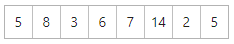#### Solution:

Standard Deviation (Actual Mean Method)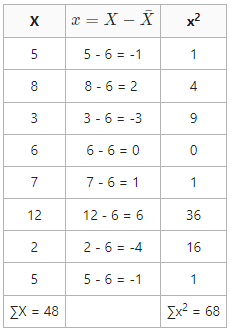Arithmetic MeanStandard Deviation (σ) =Standard Deviation = 2.9 or 3

### 2. Direct Method:

In Direct Method, there is no need to determine the deviation from the actual mean to calculate standard deviation. The steps taken to determine standard deviation through the direct method are as follows:

Step 1: First of all, calculate the actual mean (\bar{X}) of the given observations.

Step 2: Now square the observations and determine their total; i.e., ∑X2.

Step 3: Now, apply the following formula:OrWhere,

σ = Standard Deviation

∑X2 = Sum total of the squares of observations= Actual Mean

N = Number of Observations

#### Example:

Calculate the standard deviation from the following data using Direct Method: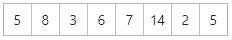#### Solution:

Standard Deviation (Direct Method)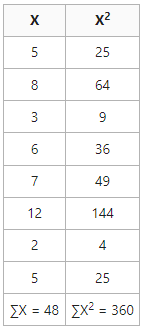Arithmetic MeanStandard Deviation (σ) =Standard Deviation = 3

### 3. Short-Cut Method (Assumed Mean Method):

Actual Mean can sometimes come in fractions which can make the calculation of standard deviation complicated and difficult. In those cases, it is suggested to use Short-Cut Method to simplify the calculations. The steps taken to determine standard deviation through the assumed mean method are as follows:

Step 1: First of all, take any value of X in the series as Assumed Mean (A).

Step 2: Now determine the deviations of the items from an assumed mean and denote the deviations by d; i.e., d = X – A.

Step 3: Calculate the sum of deviations; i.e., ∑d.

Step 4: Determine the square of the deviations and denote the total as ∑d2.

Step 5: Now, apply the following formula:Where,

σ = Standard Deviation

∑d = Sum total of deviations from assumed mean

∑d2 =  Sum total of squares of deviations

N = Number of pairs of observations

#### Example:

Calculate the standard deviation from the following data using Assumed Mean Method: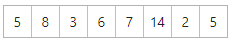#### Solution:

Standard Deviation (Assumed Mean Method)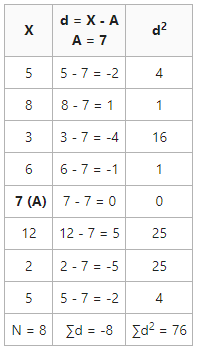Standard Deviation (σ) =Standard Deviation = 2.9 or 3

My Personal Notes arrow_drop_up